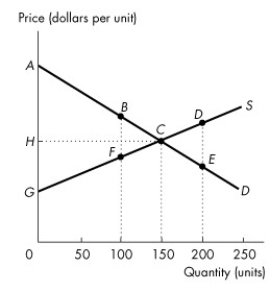# [Solved] Use the Figure Below to Answer the Following Questions

Question 85
Multiple Choice

## Use the figure below to answer the following questions.Figure 5.3.2 -Refer to Figure 5.3.2.If the level of output is 150 units, the producer surplus is area

A)BCF.
B)ACG.
C)DCE.
D)ACH.
E)HCG.

10+ million students use Quizplus to study and prepare for their homework, quizzes and exams through 20m+ questions in 300k quizzes.

Explore our library and get Economics Homework Help with various study sets and a huge amount of quizzes and questions

3.5K

Study sets

66.5K

Quizzes

5.9M

Questions

Upload material to get free accessInvite a friend and get free accessSubscribe and get an instant access